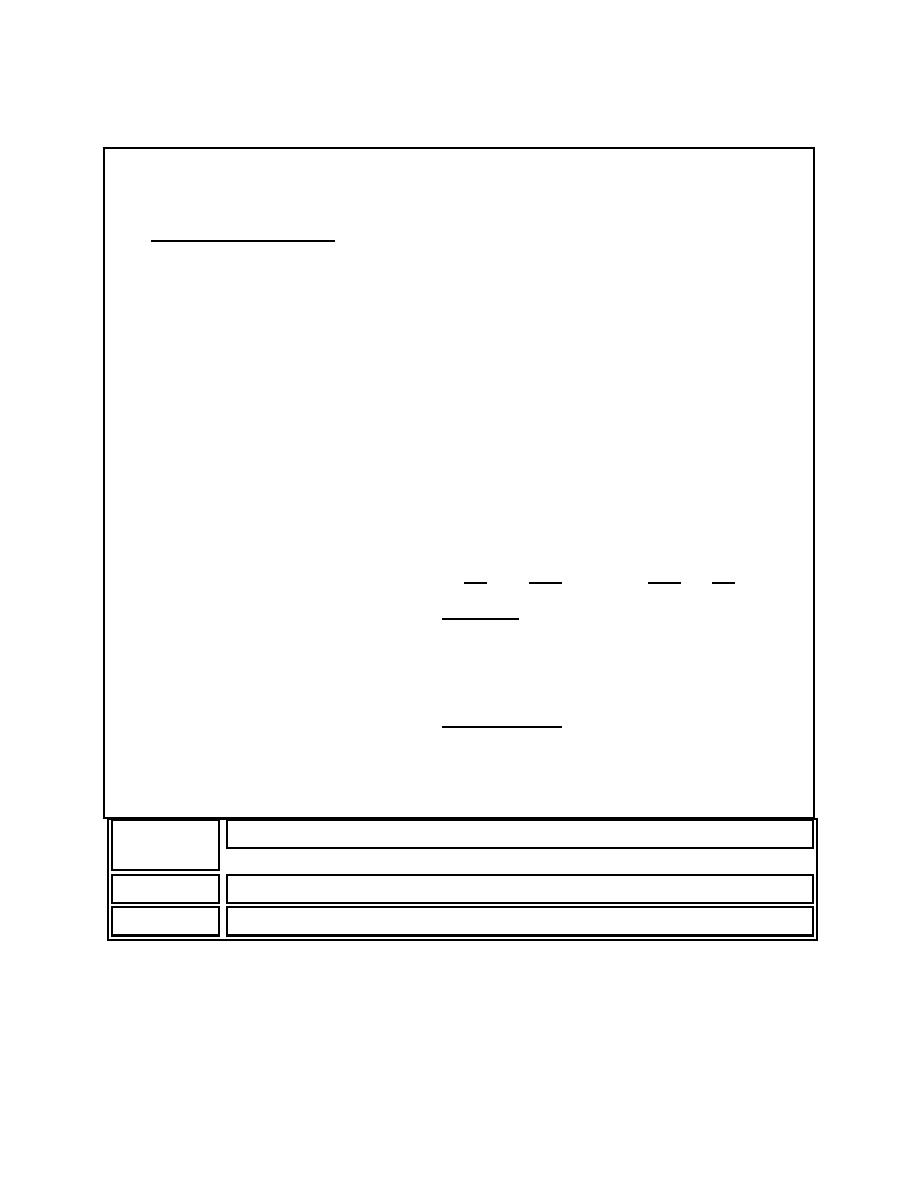Estimating in-Floor DuctCustom SearchMIL-HDBK-1012/3
_________________________________________________________________
EXAMPLE TWO
ESTIMATING IN-FLOOR DUCT
A.  Distribution Duct.  In-floor distribution duct requirements
may be estimated by applying a guideline of 6.5 sq. cm
(1 sq. in.) of duct cross-sectional area for each 10 sq. m
(100 sq. ft.) of usable floor space served.  Assuming that no
more than three cables will be pulled to each workstation and
each workstation occupies 10 sq. m (100 sq. ft.), the total
length of in-floor distribution duct may be estimated as follows:
1.
Calculate:
Length of distribution ducts = length of the area served by the
telecommunications closet (l) multiplied by the width of the same
area (w) divided by the duct separation 1.7 m (5 ft.), plus the
length of the area (l).
Note:  An additional length of the service area is added in this
calculation to account for the cost of a duct being installed
within 24 in. of both outside edges of the service area.
Length of distribution ducts = (L
xW
/5) + L
=
= (l x w) + l
5
2.  Example:
If the distribution zone is 100 ft. long and
100 ft. wide:
Length of distribution ducts = (100 x 100) + 100
5
= (10,000/5) + 100
= 2000 + 100
= 2100 ft.
Range of Cross-Sectional Area
Duct Size
Standard
21 to 25 sq. cm (3.3 to 3.9 sq. in.)
Large
49 to 57 sq. cm (7.6 to 8.9 sq. in.)
29Integrated Publishing, Inc. - A (SDVOSB) Service Disabled Veteran Owned Small Business